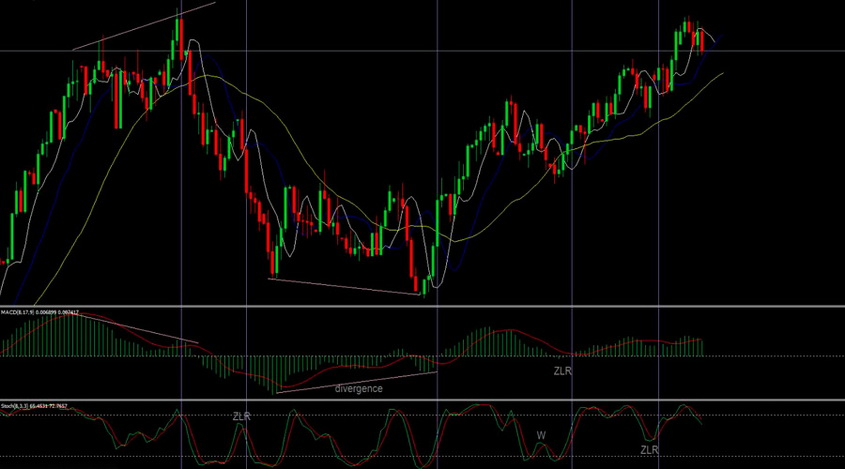# Dinapoli Levels: Application in Trade

Thursday, 3 August 2017 03:40Dinapoli levels are used in Forex trading for many years but still don’t lose their popularity among traders. The author of such a tool, J.Dinapoli, spent 35 years in technical analysis and the creation of trading strategies. He studied the Fibonacci methods and then developed his approach to the analysis of the financial market.

## Dinapoli Levels Basics

This tool is the resistance and support lines, the formation of which uses the analysis of numbers from the Fibonacci series. The author published the mathematical principle of calculation in the "Dinapoli Levels" book. The author studies many factors affecting the market and the possibility of their processing within the analysis.

To understand this method, it’s worth considering the numbers of the Fibonacci sequence in more detail.

The Fibonacci sequence has been used in mathematics for a long time. It begins with the numbers 1, 1, 2, 3, 5, 8, 13, 21. It’s easy to see that each next number of the series represents the sum of the two previous ones. In the Dinapoli analysis, the ratios of sequence numbers are used actively. For example, dividing 13 by 21, we get a number of 0.619. Division of 21 by 13 gives 1.615. If you divide 8 by 21, you get 0.281; the ratio of 21 to 8 is 2.625, and so on. Thus, the further the numbers of the sequence are located, the closer their ratio is to the figures of this sequence.

These ratios are used on the axis of the price chart, and the lines are applied to any timeframe.

### Building the Levels

The construction of the Dinapoli lines is based on the standard Fibonacci levels. This tool is based on the MetaTrader4 platform by default. However, not all the levels are applied to the chart, but only 38.2%, 50%, and 61.8%.

• Within a downtrend, the lines are built from the highest value to the lowest one;
• Within an uptrend, the lines are constructed from the minimum value to the maximum.

The range of the minimum and maximum values of an asset is called the market swing.

### Application of the Dinapoli GridThere are two critical areas in such a grid, the correction area, and the expansion one. The correction zone serves to open the transactions. The point, which the swing ends at, is called the focus point. The correction area is at this point.

A market swing can have more than one focal point. If a new focal point appears on the chart, the lines should be redrawn to reflect the new one.

When you determine the focal points and price corrections, you need to find the so-called Fibo-nodes. It’s important to see how many corrections were required to form each pair of the nodes. So it becomes possible to predict when the value of the asset will approach the node most close.

The expansion area is used to close the transactions. For such a reason, an additional grid of Fibonacci numbers is drawn, using the numbers of 261.8, 161.8 and 100. The Take Profit orders are set, and the buy or sell orders are closed right at these levels.• If bears dominate the market and a downtrend is observed, a new line is drawn from the nearest low to the correction maximum formed after the price rebound from the level of 38.2.
• Within the bullish trend, this line is drawn from the nearest maximum to the minimum of the correction, which broke through the level of 38.2.

It’s worth noting that if the value of the asset didn’t reach this line, it’s better not to draw an additional grid.

Dinapoli levels are used by many traders, but they are a quite complicated tool for the newcomers of the financial market. Their construction requires an understanding of the price dynamics principles, as well as developed mathematical skills. However, a lot of indicators were developed, based on such a system, performing the level calculations in the automatic mode.

There are a lot of trading strategies based on such levels, such as "Bonsai," "Bushes," and many others.

A risky trading style characterizes these techniques. In this case, the whole success of the trade depends entirely on the correct calculation of the lines, and their application to the chart. You follow the price corrections and calculate the Stop Loss points as a percentage of the deposit amount.

Remember that the success of the trade directly depends on the rules of the money management, and the correct risk assessment. If you want to use a new tool or a trading system for the first time, we recommend training on a demo account, before starting a real trade.